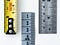# Don’t overthink Linear Regression, it is just a linear-model. In even simpler terms, a line of best fit.Photo by William Warby on Unsplash
• explain the basics of simple linear regression in ML
• use least squared error(LSE) as a measure of model quality
• make you familiar with ML jargon
• find the mathematical model of values using python, by implementing LSE and simple linear regression

## Linear Regression## Maeve B.

I'm a Junior Dev just exploring different avenues of tech